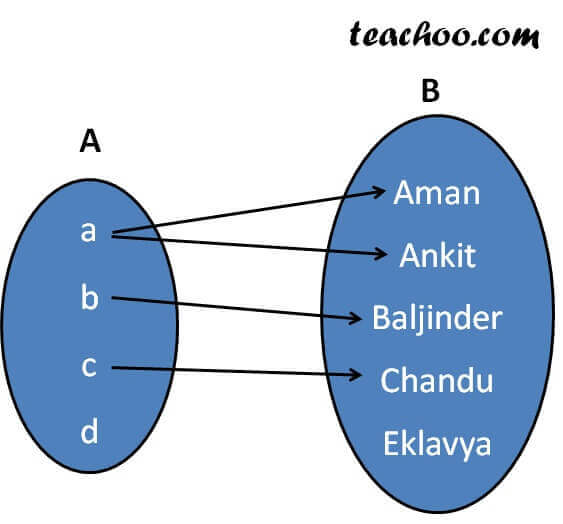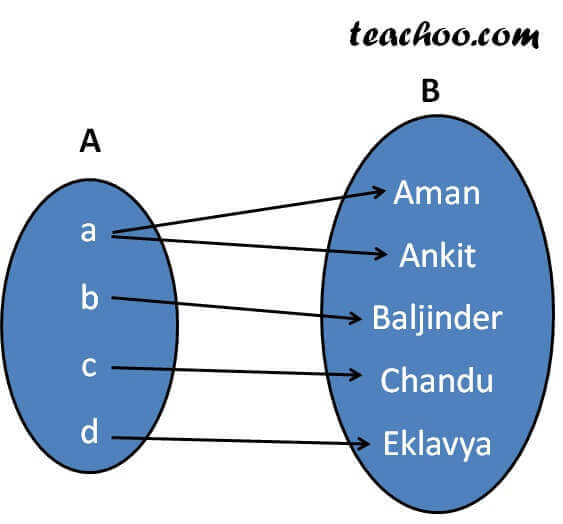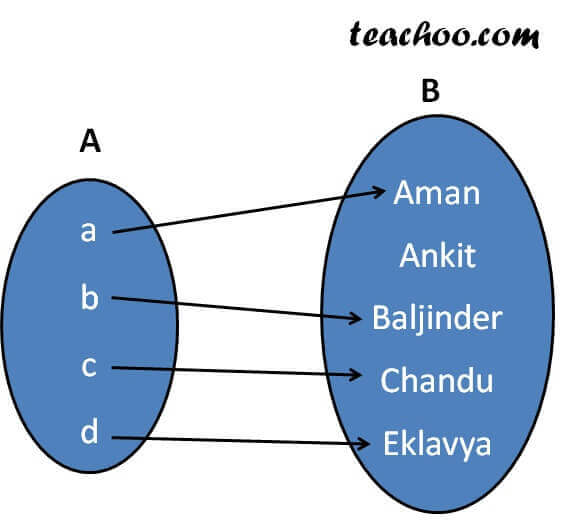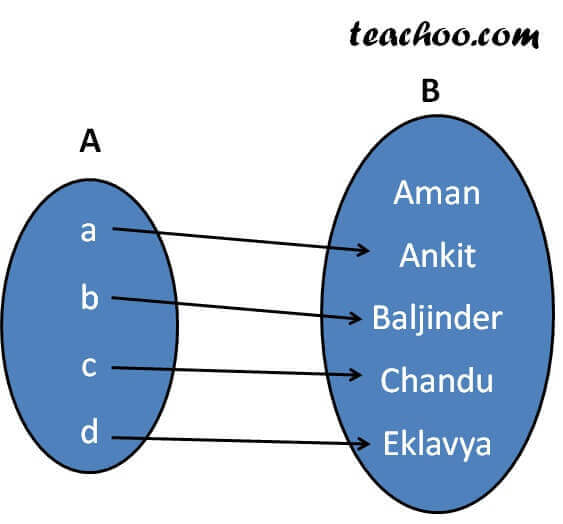Function - Definition

Chapter 1 Class 12 Relation and Functions
Concept wise

f: A -> B

A relation from A to B is a function if every element of set A has one and only one image in set B.

∴ For a function

1. Every element of set A will have an image.
2. Every element of set A will only one image in set B

Let us take an example

Let A = {a, b, c, d}

and B = {Aman, Ankit, Baljinder, Chandu, Eklavya}

## Check if the following are functions?-a-

For a function

1. Every element of set A will have an image.
2. Every element of set A will only one image in set B

Since d doesn’t have a image,

it is not a function

-ea--a-

For a function

1. Every element of set A will have an image.
2. Every element of set A will only one image in set B

Since a has two images,

it is not a function

-ea--a-

For a function

1. Every element of set A will have an image.
2. Every element of set A will only one image in set B

Since every element has an image,

and every element has only one image.

Hence, it is a function

So, the function is

f = {(a, Aman), (b, Baljinder), (c, Chandu), (d, Eklavya)}

Domain = Set of first elements = {a, b, c, d}

Range = Set of second elements = {Aman, Baljinder, Chandu, Eklavya}

Codomain = Second set = {Aman, Ankit, Baljinder, Chandu, Eklavya}

-ea-

Note : In a function, domain will always be equal to first set.-a-

For a function

1. Every element of set A will have an image.
2. Every element of set A will only one image in set B

Since every element has an image,

and every element has only one image.

Hence, it is a function

So, the function is

f = {(a, Ankit), (b, Baljinder), (c, Chandu), (d, Eklavya)}

Domain = Set of first elements = {a, b, c, d}

Range = Set of second elements = {Ankit, Baljinder, Chandu, Eklavya}

Codomain = Second set = {Aman, Ankit, Baljinder, Chandu, Eklavya}

-ea-

Solve all your doubts with Teachoo Black (new monthly pack available now!)